# Simple 2x32 Watt Audio Amplifier with TDA2050

Published  March 18, 2020   0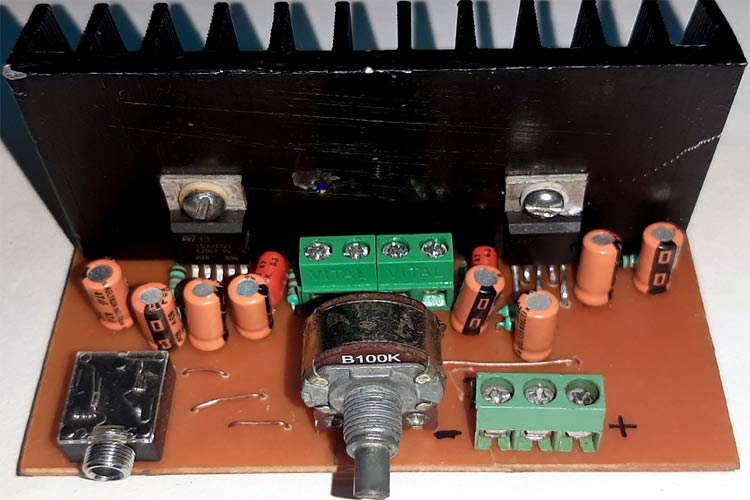If you are thinking about building a simple, cheap, and moderately high power amplifier circuit that can deliver up to 50-watt peak RMS power into a loudspeaker, then you are in the right place. In this article, we are going to use the most popular TDA2050 IC to design, demonstrate, build, and test the IC to achieve the above requirements. So without further ado, let's get started.

Also, check our other Audio amplifier circuits where we have built 25w, 40w, 100w audio amplifier circuit using op-amps, MOSFETs, and IC like IC TDA2030, TDA2040.

### Before we start

Before you start building this 32+32 Watt Audio Amplifier, you should know how much power your amplifier can deliver. Also, you need to consider the load impedance of the speaker, woofer or anything that you are building your amplifier. For more information, consider reading the datasheet.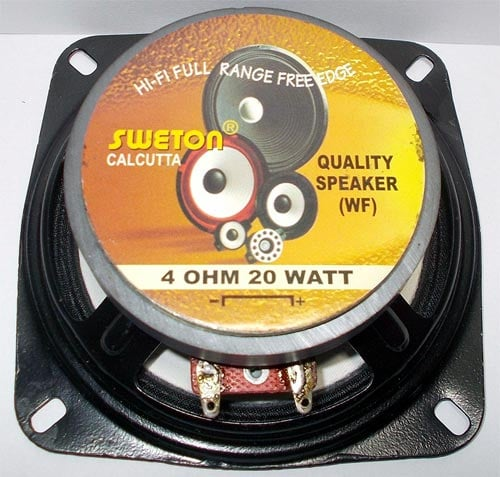By going through the datasheet, I have found that the TDA2050 can output 28 Watts into 4Ω speakers with 0.5% distortion on a 22V power supply. And I will be powering a 20-watt woofer with 4Ω impedance, which makes the TDA2050 IC a perfect choice.

### Choosing the Transformer

The sample circuit on the datasheet for the TDA2050 says that the IC can be powered from a single or a split power supply. And in this project, a dual polarity power supply will be used to power the circuit.

The goal here is to find the right transformer, which can deliver sufficient voltage and current to drive the amplifier properly.

If we consider a 12-0-12 transformer, it will output 12-0-12V AC if the input supply voltage is 230V. But as AC mains input always drifts, so the output will also drift. Taking that fact in mind, now we can calculate the supply voltage for the amplifier.

The transformer gives us AC voltage and if we convert that into DC voltage we will get-

`VsupplyDC = 12*(1.41) = 16.97VDC`

With that, it can be clearly stated that the transformer can deliver 16.97VDC when the input is 230V AC

Now if we consider voltage drift of 15%, we can see that the maximum voltage becomes-

`VmaxDC = (16.97 +2.4) = 18.97V`

Which is well within the maximum supply voltage range of the TDA2050 IC.

### Power Requirement for TDA2050 Amplifier Circuit

Now let us determine how much power will be consumed by the amplifier.

If we consider the power rating of my woofer, it is 20 watts, so a stereo amplifier will consume 20+20 = 40 watts.

Also, we have to consider the power losses and the quiescent current of the amplifier. Generally, I do not calculate all these parameters because to me it's time-consuming. So as a rule of thumb, I find the total consumed power, and multiply it by a factor of 1.3 to find out the output power.

`Pmax = (2x18.97)*1.3 = 49.32 watt`

So, to power the amplifier circuit, I am going to use a 12 - 0 - 12 transformer, with 6 Amps rating, this is a bit of overkill. But at the moment, I do not have any other transformer with me so I am going to use that.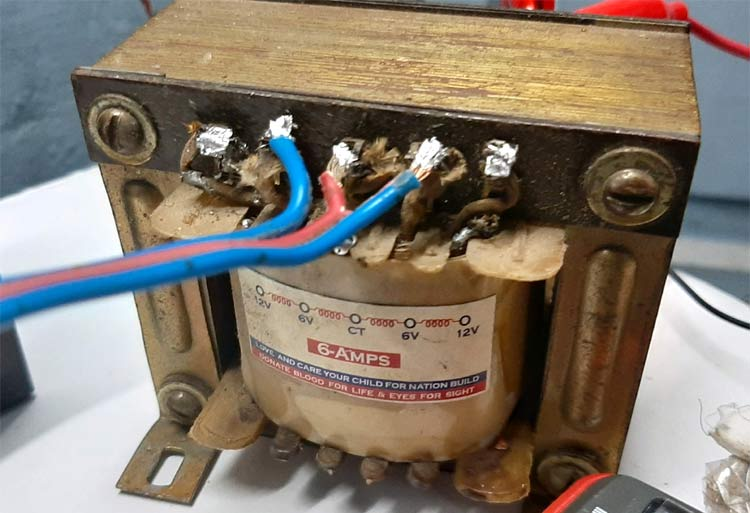### Thermal Requirements

Now, that the power requirement for this Hifi Audio Amplifier is out of the way. Let us turn our focus to finding out the thermal requirements.

For this build, I have chosen an aluminum, extrusion-type heat sink. Aluminum is a well-known substance for heat-sink because it's relatively inexpensive and exhibits good thermal performance.

To verify the maximum junction temperature of the TDA2050 IC does not exceed the maximum junction temperature, we can use the popular thermal equations, which you can find in this Wikipedia link.

We use the general principle that the temperature drop ΔT across a given absolute thermal resistance RØ with a given heat flow Q through it is.

` ΔT = Q * RØ`

Here, Q is the heat flow through the heatsink which can be written as

` Q = ΔT/RØ`

Here, ΔT is the maximum temperature drop from junction to ambient

RØ is the absolute thermal resistance.

Q is the power dissipated by the device or heat flow.

Now for the sake of the calculation, the formula can be simplified and rearranged to

`TJmax – (Tamb + ΔTHS) = Qmax * (R​ØJC + R​ØB + R​ØHA)`

Rearranging the formula

`Qmax = (TJmax – (Tamb + ΔTHS)) / (R​ØJC + R​ØB + R​ØHA)`

Here,

TJmax  is the maximum junction temperature of the device

Tamb  is the ambient air temperature

THs  is the temperature where the heatsink is attached

RØJC  is the device absolute thermal resistance from junction to case

RØB  is the typical value for an elastomer heat transfer pad for a TO-220 package

RØHA  a typical value for a heatsink for a TO-220 package

Now let's put the actual values from the datasheet of the TDA2050 IC

TJmax = 150 °C (typical for a silicon device)

Tamb = 29 °C (room temperature)

RØJC = 1.5 °C/W (for a typical TO-220 package)

RØB = 0.1 °C/W (typical value for for an elastomer heat transfer pad for a TO-220 package)

RØHA = 4 °C/W (a typical value for a heatsink for a TO-220 package)

So, the final result becomes

`Q = (150 - 29) / (1.5+0.1+4) = 17.14W`

This means we have to dissipate 17.17 watts or more to prevent the device from overheating and getting damaged.

### Setting the Gain

Setting up the gain for the amplifier is the most important step of the build, as a low gain setting may not provide enough power. And a high gain setting will certainly distort the amplified output signal of the circuit. With my experience, I can tell that a gain setting from 30 to 35 dB is good for playing audio with a smartphone or a USB audio kit.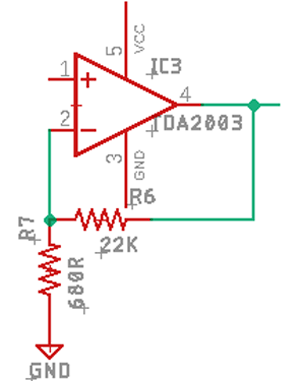The example circuit in the datasheet recommends a gain setting of 32db and I am going to just leave it as it is.

The gain of the Op-Amp can be calculated by the following formula

```AV = 1+(R6/R7)
AV = 1+(22000/680) = 32.3db```

Which works just fine for this amplifier

Note: For setting up the amplifiers gain 1% or 0.5% resistors must be used otherwise the stereo channels will produce different outputs

### Setting up The Input Filter for the Amplifier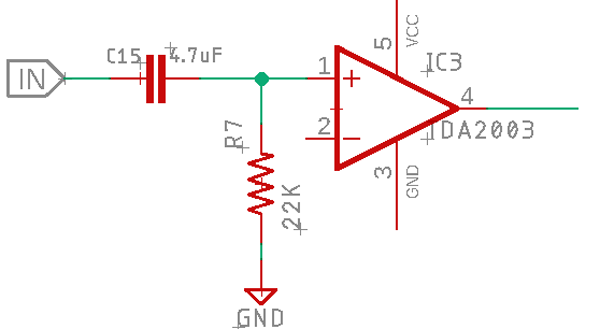The capacitor C1 acts as a DC blocking capacitor thus reduces noise.

The Capacitor C1 and the resistor R7 create an RC high pass filter, which determines the lower end of the bandwidth.

The cutoff frequency of the amplifier can be found by using the following formula shown below.

`FC = 1 / (2πRC)`

Where R and C are the values of the components.

To find the values of the C, we have to rearrange the equation to:

`C = 1 / (2π x 22000R x 3.5Hz) = 4.7uF`

Note: It is recommended to use metal film oil capacitors for the best audio performance.

### Setting up The Bandwidth in the Feedback Loop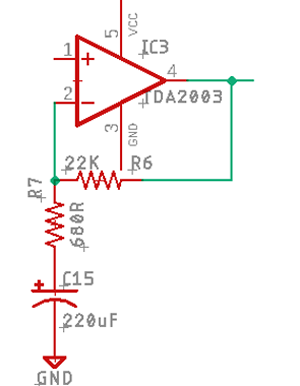The capacitor in the feedback loop helps to make a low pass filter, which helps to enhance the bass response of the amplifier. The smaller the value of the C15, the softer the bass will get. And a larger value for C15 will give you a more punchy bass.

### Setting the Output Filter

An output filter or commonly known as a Zobel network prevents oscillations generated from the speaker coil and wires. It also rejects the radio interference which is picked up by the long wire from the speaker to the amplifier; it also prevents them from going into the feedback loop.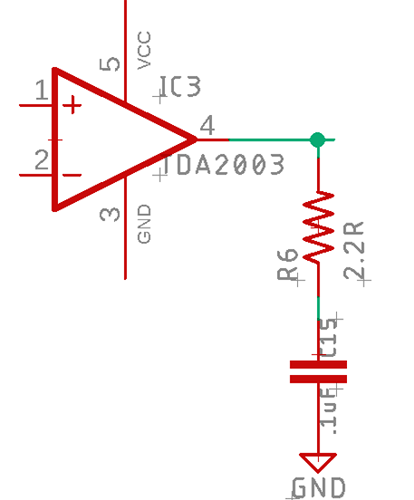The cutoff frequency of the Zobel network can be calculated by the following simple formula

The datasheet gives values for the R and C, which is R6 = 2.2R and C15 = 0.1uF If we put the values in the formula and calculate we will get a cut-off frequency of

```Fc = 1 / (2π x 2.2 x (1 x 10^-7))
= 723 kHz```

723 kHz is above the human hearing range of 20 kHz, so it will not affect the output frequency response and it will also prevent wired noise and oscillations.

### The Power Supply

A dual polarity power supply with proper decoupling capacitors is required to power the amplifier, and the schematic is shown below.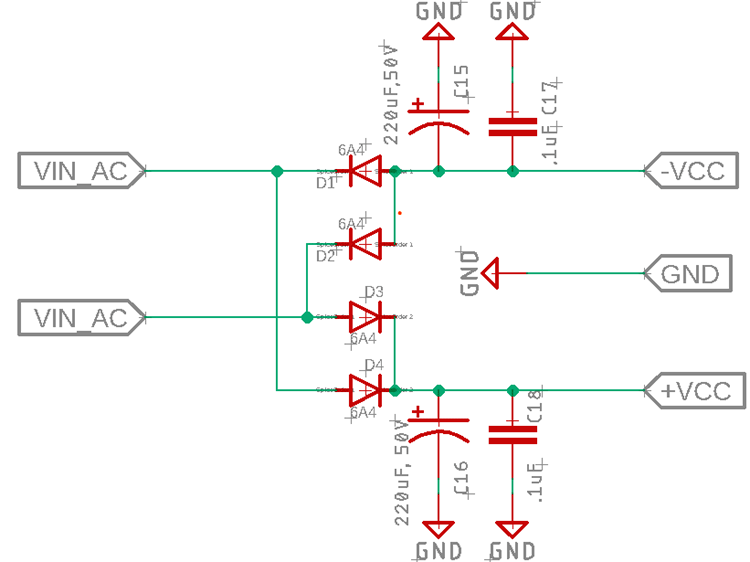### Components Required

• TDA2050 IC - 2
• 100k Variable Pot - 1
• Screw Terminal 5mmx2 - 2
• Screw Terminal 5mmx3 - 1
• 0.1µF Capacitor - 6
• 22k Ohms Resistor - 4
• 2.2 Ohm Resistor - 2
• 1k Ohm Resistor - 2
• 47µF Capacitor - 2
• 220µF Capacitor - 2
• 2.2µF Capacitor - 2
• 3.5mm Headphone Jack - 1
• Clad Board 50x 50mm - 1
• Heat Sink - 1
• 6Amp Diode - 4
• 2200µF Capacitor - 2

### The Schematic

Circuit diagram for the TDA2050 amplifier circuit is given below: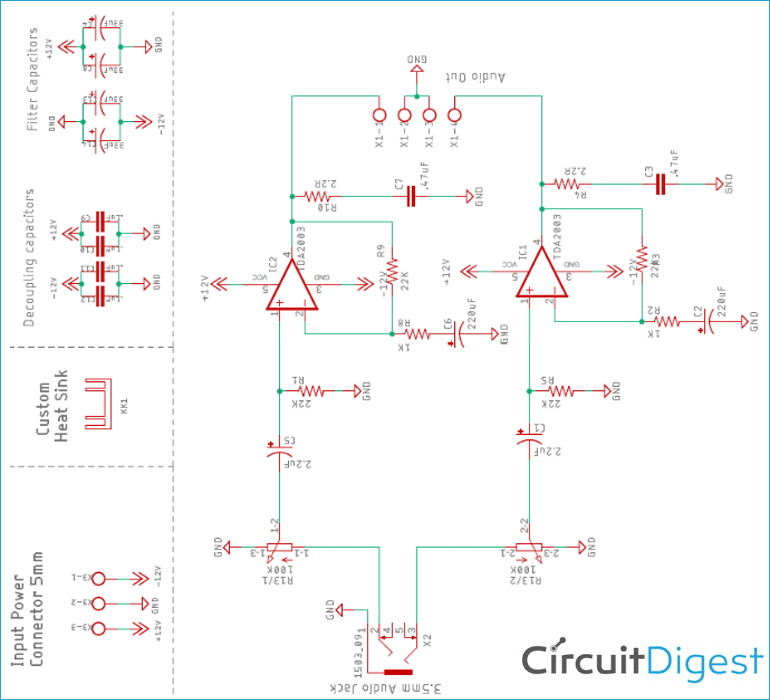### Circuit Construction

For the demonstration of this 32-watt power amplifier, the circuit is constructed on a handmade PCB with the help of the schematic and PCB design files. Please note that if we are connecting a big load to the output of the amplifier, a huge amount of current will flow through the PCB traces, and there's a chance that the traces will burn out. So, to prevent the PCB traces from burning out, I have included some jumpers which help to increase the current flow.

### Testing the TDA2050 Amplifier Circuit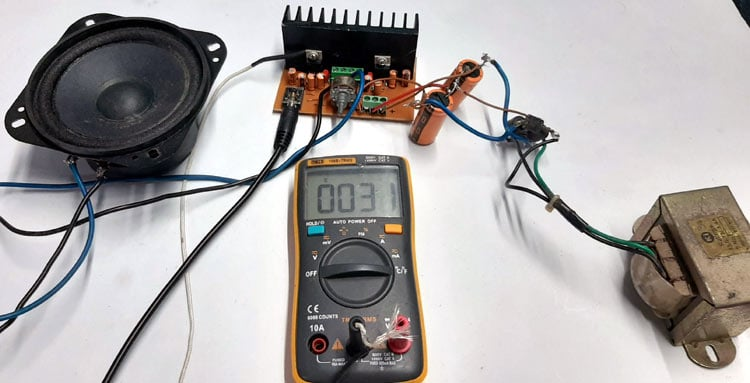To test the circuit, the following apparatus was used.

1. A transformer which has a 13-0-13 Tap
2. A 4Ω 20W speaker as a load
3. Meco 108B+TRMS Multimeter as the temperature sensor
4. And my Samsung phone as an audio source

As you can see above, I have mounted the temperature sensor of the multimeter directly to the heat sink of the IC to measure the temperature of the IC during the time of the testing.

Also, you can see the room temperature was 31 °C during the time of testing. At this moment, the amplifier was in an off state and the multimeter was just showing the room temperature. At the time of testing, I have added some salt in the woofer cone to show you the bass, it producing in this circuit the bass will be low because I didn't use a tone control circuit to boost the bass. I am going to do that in the next article.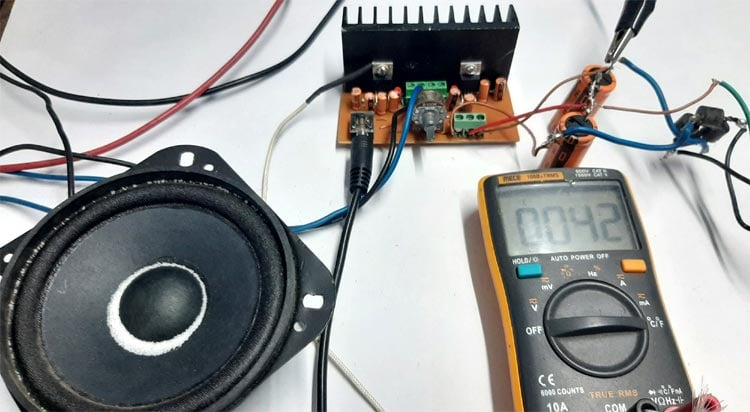You can see from the above image, the results were more or less great and the temperature of the IC didn't go beyond 50 °C during the testing.

### Further Enhancement

The circuit can be further modified in order to improve its performance like we can add an additional filter in order to reject high-frequency noises. The size of the heat sink needs to be bigger in order to reach a full load condition of 32W. But that is a subject for another project which is coming soon by the way.

I hope you liked this article and learned something new out of it. If you have any doubt, you can ask in the comments below or can use our forums for detailed discussion.

Also, check our other audio amplifier circuits.

Video